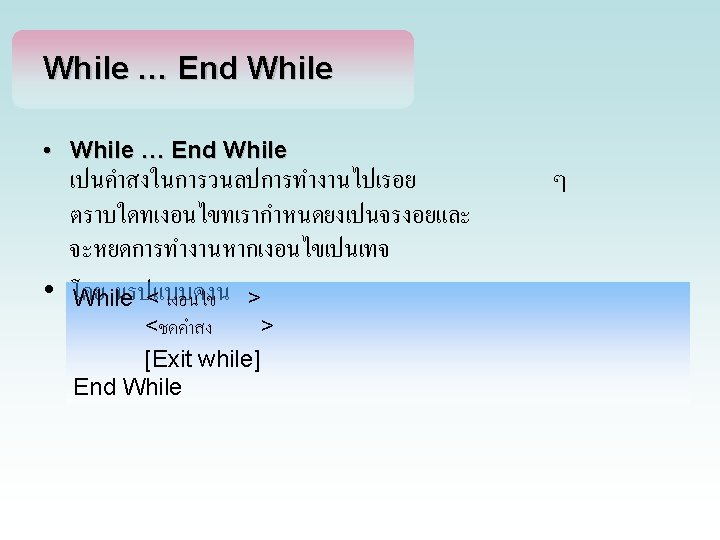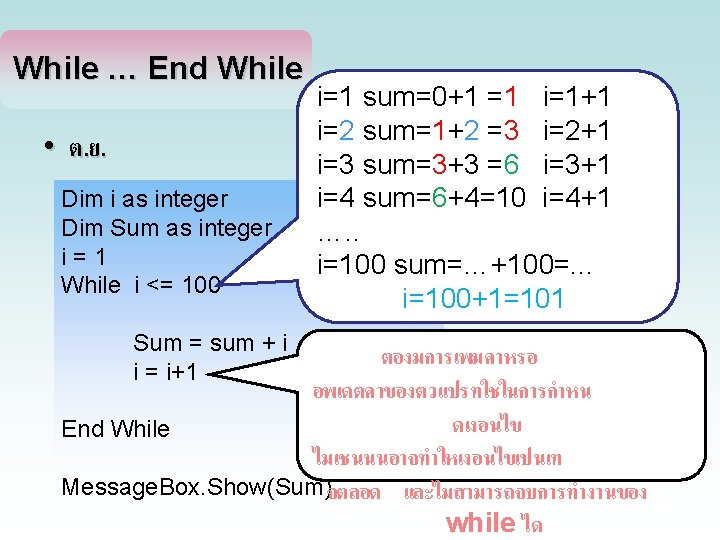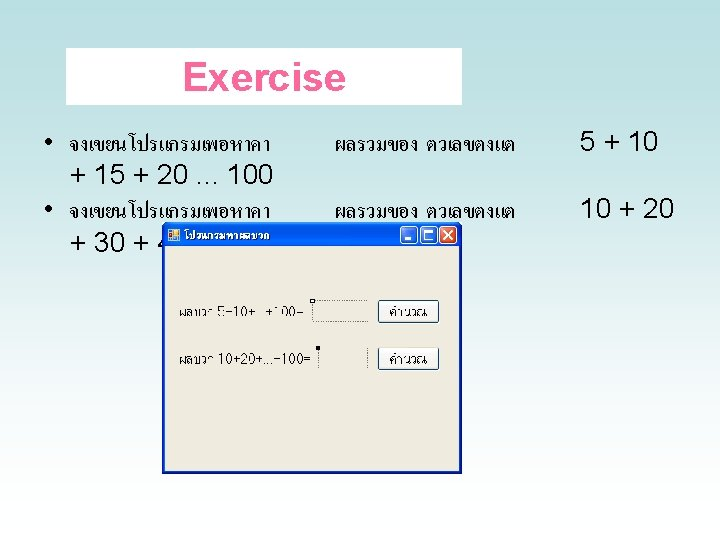# IF Then Else IF Dim Score as Integer

• Slides: 18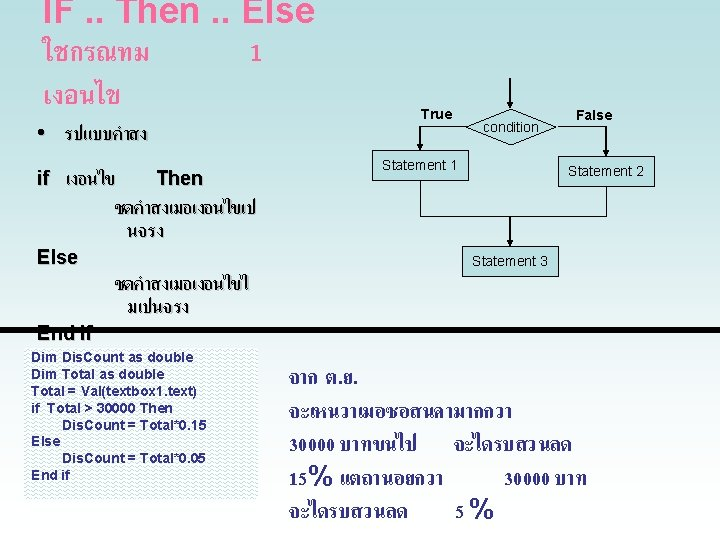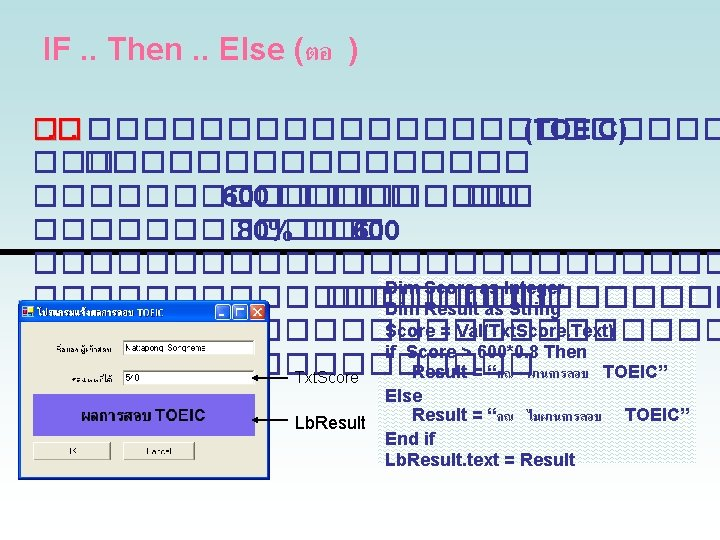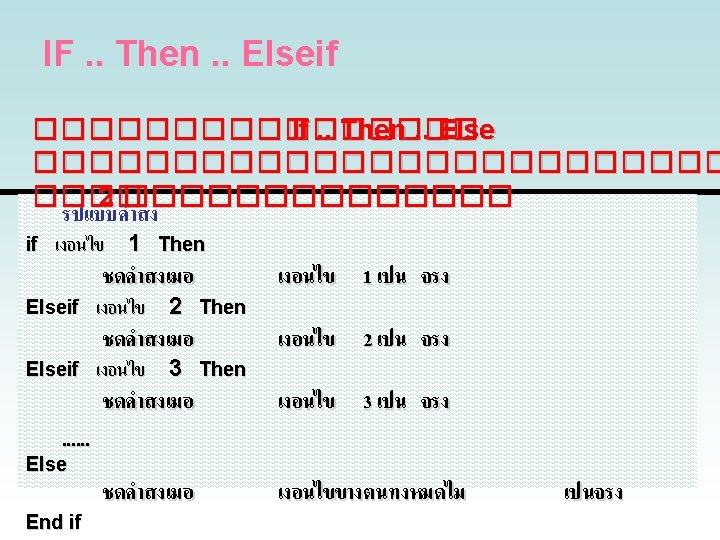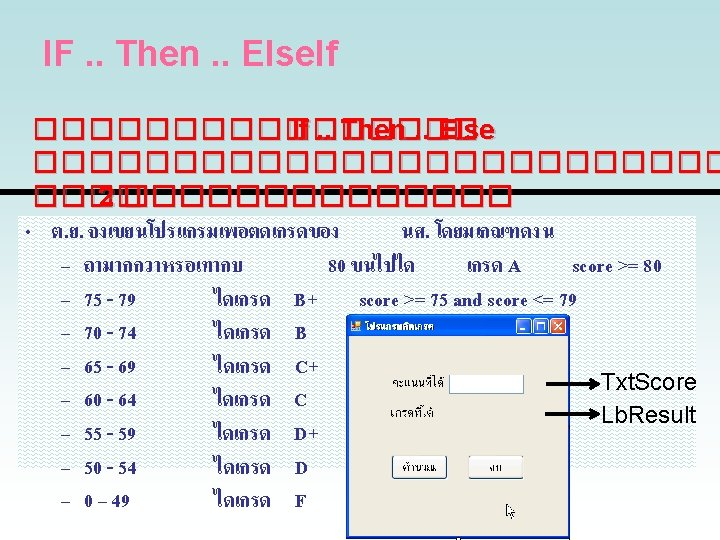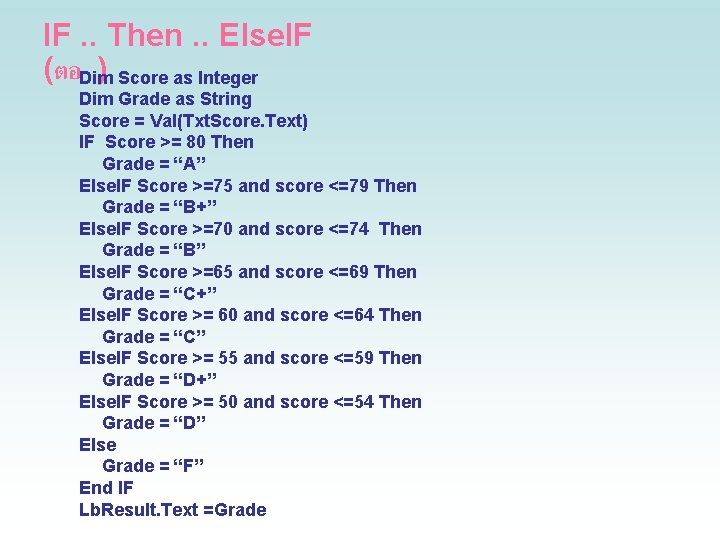IF. . Then. . Else. IF (ตอDim) Score as Integer Dim Grade as String Score = Val(Txt. Score. Text) IF Score >= 80 Then Grade = “A” Else. IF Score >=75 and score <=79 Then Grade = “B+” Else. IF Score >=70 and score <=74 Then Grade = “B” Else. IF Score >=65 and score <=69 Then Grade = “C+” Else. IF Score >= 60 and score <=64 Then Grade = “C” Else. IF Score >= 55 and score <=59 Then Grade = “D+” Else. IF Score >= 50 and score <=54 Then Grade = “D” Else Grade = “F” End IF Lb. Result. Text =Grade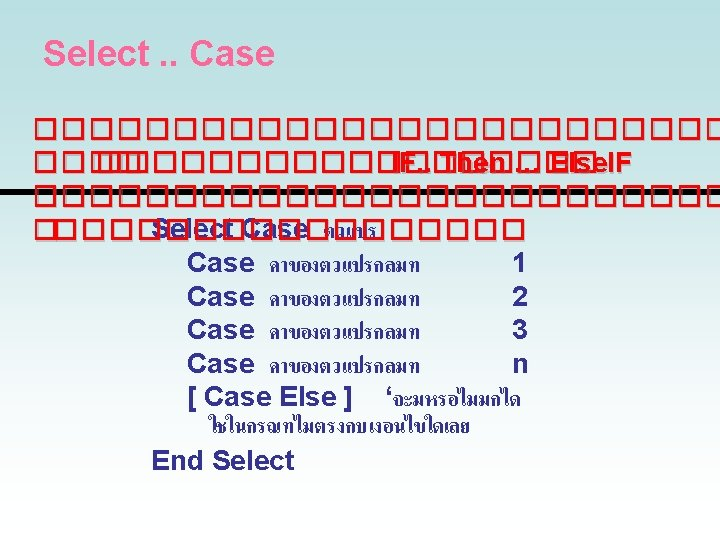Select. . Case (ตอ ) �. �. ������������ �. �. ������ Select Case Dim score As Integer Dim Grade As String score = Txt. Score. Text Select Case Val(score) Case Is >= 80 Grade = "A" Case 75 To 79 Grade = "B+" Case 70 To 74 Grade = "B" Case 65 To 69 Grade = "C+" Case 60 To 64 Grade = "C" Case 55 To 59 Grade = "D+" Case 50 To 54 Grade = "D" Case 0 To 49 Grade = “F" End Select Lb. Result. Text = Grade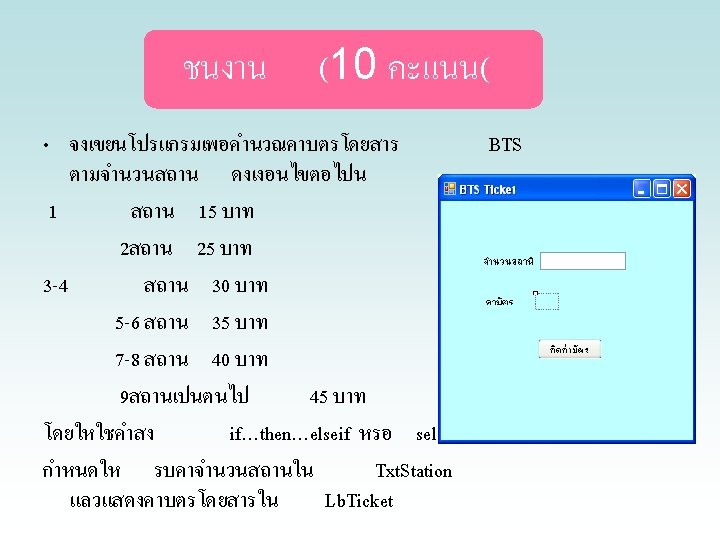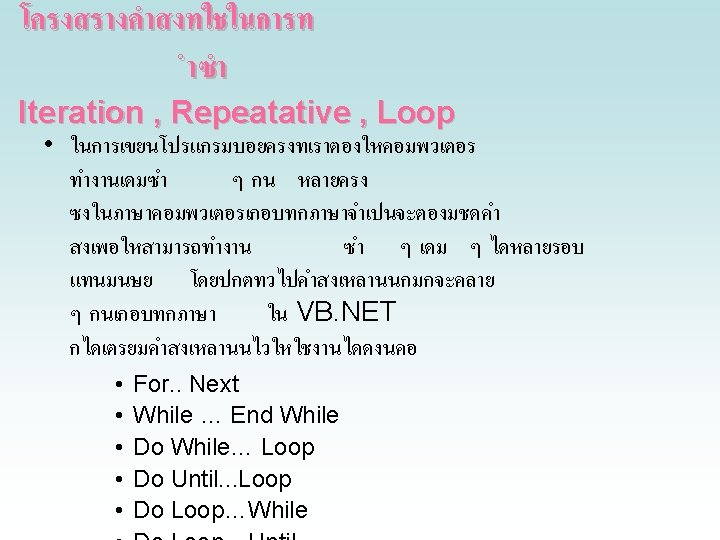For … Next • ต. ย. จงเขยนโปรแกรมเพอหาคา. . . 100 ผลรวมของ 1 + 2 + 3 Dim i as integer Dim Sum as integer For i = 1 to 100 Sum = Sum + i Next i Label 1. Text = Sum i=1 sum=0+1 =1 i=2 sum=1+2 =3 i=3 sum=3+3 = 6 i=4 sum=6+4=10 …. . i=100 sum=…+100=. . .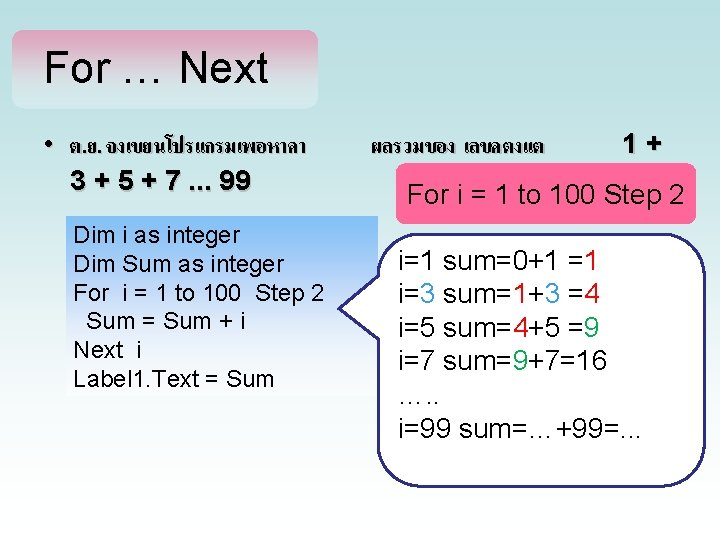For … Next • ต. ย. จงเขยนโปรแกรมเพอหาคา 3 + 5 + 7. . . 99 Dim i as integer Dim Sum as integer For i = 1 to 100 Step 2 Sum = Sum + i Next i Label 1. Text = Sum ผลรวมของ เลขคตงแต 1+ For i = 1 to 100 Step 2 i=1 sum=0+1 =1 i=3 sum=1+3 =4 i=5 sum=4+5 =9 i=7 sum=9+7=16 …. . i=99 sum=…+99=. . .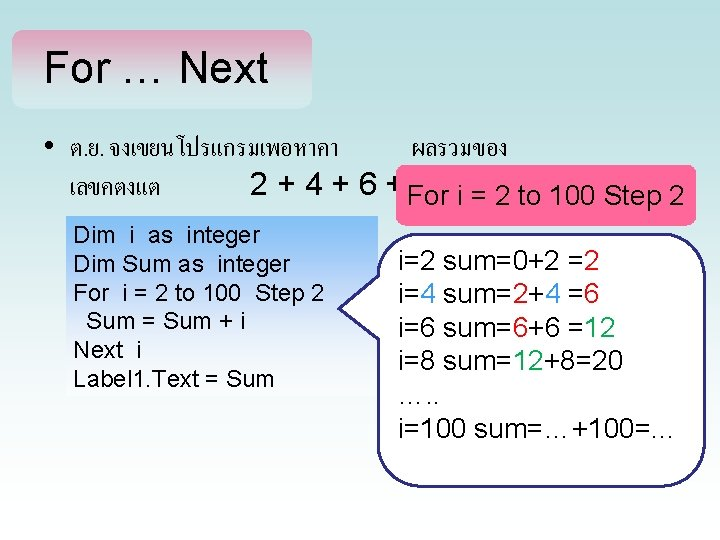For … Next • ต. ย. จงเขยนโปรแกรมเพอหาคา ผลรวมของ เลขคตงแต 2 + 4 + 6 + For 8. . . i =100 2 to 100 Step 2 Dim i as integer Dim Sum as integer For i = 2 to 100 Step 2 Sum = Sum + i Next i Label 1. Text = Sum i=2 sum=0+2 =2 i=4 sum=2+4 =6 i=6 sum=6+6 =12 i=8 sum=12+8=20 …. . i=100 sum=…+100=. . .